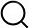# Also in the Article

Stability analysis
This protocol is extracted from research article:
Coherent perfect absorption of nonlinear matter waves

Procedure

We analyzed the stability for a BEC within the framework of the discrete model(6)and required that the left and right incident superfluid currents have to be stable. Their stability is determined by the stability of the corresponding Bogoliubov phonons on an infinite homogeneous lattice (that is, without applied removal of atoms). The stability of the homogeneous lattice is found using the substitution(7)where ρ > 0 characterizes the uniform density, and |vn| , |wn| ≪ ρ are small perturbations. Linearizing Eq. 6 (with γ = 0) with respect to vn and wn, we found two dispersion branches(8)Consider now a positive scattering length, U > 0, which corresponds to the experiments reported here. One can then identify the stability domain for Bogoliubov phonons and, hence, the stability of the superfluid current, requiring ω± to be real for the given q and all real k. This results in the constraint 0 ≤ q < π/2, that is, only slow currents are dynamically stable.

Note: The content above has been extracted from a research article, so it may not display correctly.

Q&A